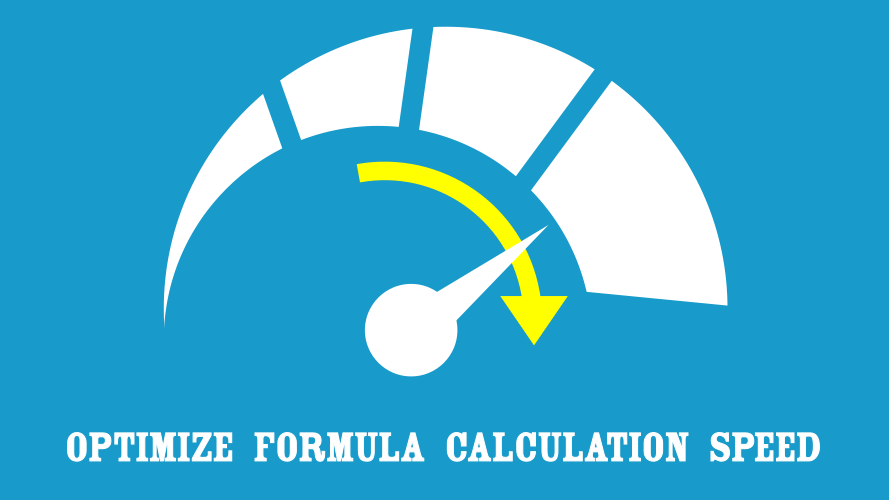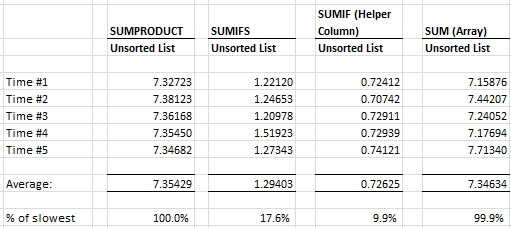# Optimize Formula Calculation Speed – Part 8: Sum with multiple criteria## Part 8 – Sum with multiple criteria

In the previous part, we saw that helper columns (a column which stores intermediate results to be used in other calculations) create significantly faster calculations times when used with a lookup. SUMIFS is our next fastest option (this only works when used to return numbers).

The INDEX/MATCH, SUMPRODUCT, SUM (Array) formulas were all a similar speed.  However, of those only INDEX/MATCH will work as a non-numerical lookup, so is probably the best option in that circumstances.

In this part, we will be considering non-unique data, specifically how to SUM using multiple criteria. You can download the workbook here.

## Setting up the test

Our original dataset for this series had 99,999 lines, it is this dataset which will be using for our test.  From the 99,999 lines, I had selected 100 lines at random.  I carried out 5 timed tests on each formula using the MircoTimer to measure how long the 100 lines took to recalculate.

The formulas we will be considering are:

SUMPRODUCT

SUMIFS

SUMIF (Helper Column)

SUM (array)

## The results

The results are in the table below.Once again, SUMPRODUCT is the slowest formula. It has been included in all our tests, as it is a very flexible, but this flexibility comes at the cost of speed.

SUM (Array) achieves a similar speed to SUMPRODUCT. They are effectively following the same logic in their calculation method, so as expected they achieve similar times.

SUMIFS is a much faster calculation, being able to achieve the same result in just 18% of the time of SUMPRODUCT.  SUMIFS was added in Excel 2007.  It was designed specifically for summing across large datasets with multiple criteria.  Incidentally, the COUNTIFS and AVERAGEIFS formulas were also introduced at the same time, and are also reasonably fast formulas.

The fastest result, just like the previous part, is when a helper column is used. In our example, we have used the helper column with SUMIF.  The helper column reduces calculation time to just 10% of SUMPRODUCT.

Just as a note, if we use SUMIFS with the helper column it is approximately 3% slower than SUMIF with a helper column.  It appears that it is certainly the helper column, rather than the formula which is resulting in the speed benefit.  With such a small performance difference between SUMIFS and SUMIF you may decide to banish SUMIF from your formula knowledge altogether; deciding just to use SUMIFS going forward.

## Conclusion

Helper columns win the day again – they are great calculation time savers. Where helper columns are not suitable (which often they are not), SUMIFS is the best option.

## Next time

In the next part, we will be considering a number of other factors which can have an impact on speed.  For example, how much impact does having the data in a separate workbook have?  Or how much slower is a laptop when running on battery power